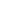## Croissance des tissus mous

### Publications sur ce thème (en anglais)

• Petal shapes of sympetaleous flowers: the interplay between growth, geometry and elasticity
Martine Ben Amar, Martin Michael Müller, Miguel Trejo

The growth of a thin elastic sheet imposes constraints on its geometry such as its Gaussian curvature KG. In this paper, we construct the shapes of sympetalous bell-shaped flowers with a constant Gaussian curvature. Minimizing the bending energies of both the petal and the veins, we are able to predict quantitatively the global shape of these flowers. We discuss two toy problems where the Gaussian curvature is either negative or positive. In the former case the axisymmetric pseudosphere turns out to mimic the correct shape before edge curling; in the latter case, singularities of the mathematical surface coincide with strong veins. Using a variational minimization of the elastic energy, we find that the optimal number for the veins is either four, five or six, a number which is deceptively close to the statistics on real flowers in nature.

New J. Phys., 14: 085014, 2012. Choisi pour les Highlights of 2012.

• Self-Contact and Instabilities in the Anisotropic Growth of Elastic Membranes
Norbert Stoop, Falk K. Wittel, Martine Ben Amar, Martin Michael Müller, Hans J. Herrmann

We investigate the morphology of thin discs and rings growing in circumferential direction. Recent analytical results suggest that this growth produces symmetric excess cones (e-cones). We study the stability of such solutions considering self-contact and bending stress. We show that, contrary to what was assumed in previous analytical solutions, beyond a critical growth factor, no symmetric e-cone solution is energetically minimal any more. Instead, we obtain skewed e-cone solutions having lower energy, characterized by a skewness angle and repetitive spiral winding with increasing growth. These results are generalized to discs with varying thickness and rings with holes of different radii.

Phys. Rev. Lett., 105(6): 068101, 2010. Cf. aussi arXiv:1007.1871.

• Hamiltonian formulation of surfaces with constant Gaussian curvature
Miguel Trejo, Martine Ben Amar, Martin Michael Müller

Dirac's method for constrained Hamiltonian systems is used to describe surfaces of constant Gaussian curvature. A geometrical free energy, for which these surfaces are equilibrium states, is introduced and interpreted as an action. An equilibrium surface can then be generated by the evolution of a closed space curve. Since the underlying action depends on second derivatives, the velocity of the curve and its conjugate momentum must be included in the set of phase space variables. Furthermore, the action is linear in the acceleration of the curve and possesses a local symmetry---reparametrization invariance---which implies primary constraints in the canonical formalism. These constraints are incorporated into the Hamiltonian through Lagrange multiplier functions, that are identified as the components of the acceleration of the curve. The formulation leads to four first order partial differential equations, one for each canonical variable. With the appropriate choice of parametrization only one of these equations has to be solved to obtain the surface which is swept out by the evolving space curve. To illustrate the formalism, several evolutions of pseudospherical surfaces are discussed.

J. Phys. A: Math. Theor., 42(42): 425204, 2009.

• Conical Defects in Growing Sheets
Martin Michael Müller, Martine Ben Amar, Jemal Guven

A growing or shrinking disc will adopt a conical shape, its intrinsic geometry characterized by a surplus angle φe at the apex. If growth is slow, the cone will find its equilibrium. Whereas this is trivial if φe≤0, the disc can fold into one of a discrete infinite number of states if φe is positive. We construct these states in the regime where bending dominates, determine their energies and how stress is distributed in them. For each state a critical value of φe is identified beyond which the cone touches itself. Before this occurs, all states are stable; the ground state has twofold symmetry.

Phys. Rev. Lett., 101(15): 156104, 2008. Cf. aussi arXiv:0807.1814.